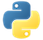# GroupByPydoc

Takes a collection of elements and produces a collection grouped, by properties of those elements.

Unlike `GroupByKey`, the key is dynamically created from the elements themselves.

## Grouping Examples

In the following example, we create a pipeline with a `PCollection` of fruits.

We use `GroupBy` to group all fruits by the first letter of their name.

``````with beam.Pipeline() as p:
grouped = (
p
| beam.Create(['strawberry', 'raspberry', 'blueberry', 'blackberry', 'banana'])
| beam.GroupBy(lambda s: s))``````

Output:

``````('s', ['strawberry']),
('r', ['raspberry']),
('b', ['banana', 'blackberry', 'blueberry']),``````View source code

We can group by a composite key consisting of multiple properties if desired.

``````with beam.Pipeline() as p:
grouped = (
p
| beam.Create(['strawberry', 'raspberry', 'blueberry', 'blackberry', 'banana'])
| beam.GroupBy(letter=lambda s: s, is_berry=lambda s: 'berry' in s))``````

The resulting key is a named tuple with the two requested attributes, and the values are grouped accordingly.

Output:

``````(NamedTuple(letter='s', is_berry=True), ['strawberry']),
(NamedTuple(letter='r', is_berry=True), ['raspberry']),
(NamedTuple(letter='b', is_berry=True), ['blackberry', 'blueberry']),
(NamedTuple(letter='b', is_berry=False), ['banana']),``````View source code

In the case that the property one wishes to group by is an attribute, a string may be passed to `GroupBy` in the place of a callable expression. For example, suppose I have the following data

``````GROCERY_LIST = [
beam.Row(recipe='pie', fruit='strawberry', quantity=3, unit_price=1.50),
beam.Row(recipe='pie', fruit='raspberry', quantity=1, unit_price=3.50),
beam.Row(recipe='pie', fruit='blackberry', quantity=1, unit_price=4.00),
beam.Row(recipe='pie', fruit='blueberry', quantity=1, unit_price=2.00),
beam.Row(recipe='muffin', fruit='blueberry', quantity=2, unit_price=2.00),
beam.Row(recipe='muffin', fruit='banana', quantity=3, unit_price=1.00),
]``````

We can then do

``````with beam.Pipeline() as p:
grouped = p | beam.Create(GROCERY_LIST) | beam.GroupBy('recipe')``````

Output:

``````('pie',
[
beam.Row(recipe='pie', fruit='strawberry', quantity=3, unit_price=1.50),
beam.Row(recipe='pie', fruit='raspberry', quantity=1, unit_price=3.50),
beam.Row(recipe='pie', fruit='blackberry', quantity=1, unit_price=4.00),
beam.Row(recipe='pie', fruit='blueberry', quantity=1, unit_price=2.00),
]),
('muffin',
[
beam.Row(recipe='muffin', fruit='blueberry', quantity=2, unit_price=2.00),
beam.Row(recipe='muffin', fruit='banana', quantity=3, unit_price=1.00),
]),``````View source code

It is possible to mix and match attributes and expressions, for example

``````with beam.Pipeline() as p:
grouped = (
p | beam.Create(GROCERY_LIST)
| beam.GroupBy('recipe', is_berry=lambda x: 'berry' in x.fruit))``````

Output:

``````(NamedTuple(recipe='pie', is_berry=True),
[
beam.Row(recipe='pie', fruit='strawberry', quantity=3, unit_price=1.50),
beam.Row(recipe='pie', fruit='raspberry', quantity=1, unit_price=3.50),
beam.Row(recipe='pie', fruit='blackberry', quantity=1, unit_price=4.00),
beam.Row(recipe='pie', fruit='blueberry', quantity=1, unit_price=2.00),
]),
(NamedTuple(recipe='muffin', is_berry=True),
[
beam.Row(recipe='muffin', fruit='blueberry', quantity=2, unit_price=2.00),
]),
(NamedTuple(recipe='muffin', is_berry=False),
[
beam.Row(recipe='muffin', fruit='banana', quantity=3, unit_price=1.00),
]),``````View source code

.

## Aggregation

Grouping is often used in conjunction with aggregation, and the `aggregate_field` method of the `GroupBy` transform can be used to accomplish this easily. This method takes three parameters: the field (or expression) which to aggregate, the `CombineFn` (or associative `callable`) with which to aggregate by, and finally a field name in which to store the result. For example, suppose one wanted to compute the amount of each fruit to buy. One could write

``````with beam.Pipeline() as p:
grouped = (
p
| beam.Create(GROCERY_LIST)
| beam.GroupBy('fruit')
.aggregate_field('quantity', sum, 'total_quantity'))``````

Output:

``````NamedTuple(fruit='strawberry', total_quantity=3),
NamedTuple(fruit='raspberry', total_quantity=1),
NamedTuple(fruit='blackberry', total_quantity=1),
NamedTuple(fruit='blueberry', total_quantity=3),
NamedTuple(fruit='banana', total_quantity=3),``````View source code

.

Similar to the parameters in `GroupBy`, one can also aggregate multiple fields and by expressions.

``````with beam.Pipeline() as p:
grouped = (
p
| beam.Create(GROCERY_LIST)
| beam.GroupBy('recipe')
.aggregate_field('quantity', sum, 'total_quantity')
.aggregate_field(lambda x: x.quantity * x.unit_price, sum, 'price'))``````

Output:

``````NamedTuple(recipe='pie', total_quantity=6, price=14.00),
NamedTuple(recipe='muffin', total_quantity=5, price=7.00),``````View source code

.

One can, of course, aggregate the same field multiple times as well. This example also illustrates a global grouping, as the grouping key is empty.

``````with beam.Pipeline() as p:
grouped = (
p
| beam.Create(GROCERY_LIST)
| beam.GroupBy()
.aggregate_field('unit_price', min, 'min_price')
.aggregate_field('unit_price', MeanCombineFn(), 'mean_price')
.aggregate_field('unit_price', max, 'max_price'))``````

Output:

``NamedTuple(min_price=1.00, mean_price=7 / 3, max_price=4.00),``View source code

.Pydoc